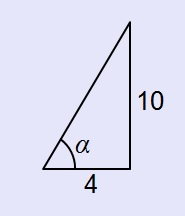Home > CCA2 > Chapter 9 > Lesson 9.1.2 > Problem9-30

9-30.
1.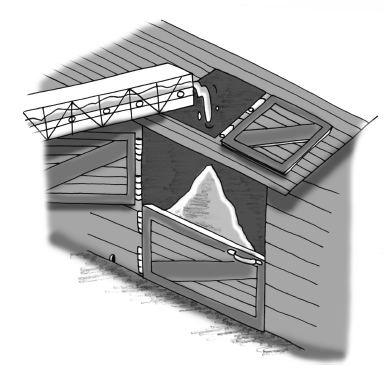A conveyor belt carries grain to the top of a barn, where it drops the grain so that it forms a pile on the ground. The pile is in the shape of a cone. Recall that the volume of a cone is one-third the area of the base times the vertical height. Homework Help ✎

1. Find the volume of the grain when the height of the pile is ten feet and the radius of the base is 4 feet. Use appropriate precision on your results.

2. As the pile grows, the cones formed remain similar. If the radius of the base is four feet when the height is ten feet, what is the radius of the base when the height is 15 feet?

3. Consider the angle the side of the cone-shaped pile makes with the ground. Is this angle changing? Justify your answer. If not, what is the measure of the angle?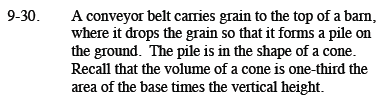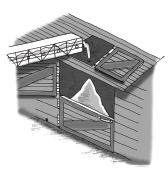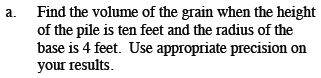$V = \frac{1}{3}(\pi r^{2})h$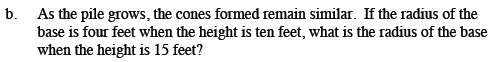Write a proportional equation and solve for the unknown value.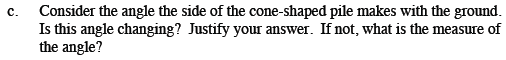What trig function would help you find the angle?